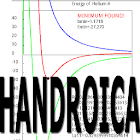All Android applications categories

All Android games categories# HANDROICA

6 9.6

9.6 Users
rating

## Screenshots

Description

HANDROICA is an anagram of "Hadronica", the Spanish word for
as protons and neutrons. Hadronic physics is a branch of Nuclear and
Particle Physics that describes the structure and interactions of
Hadrons. The principles of Quantum Physics and Quantum Field Theory
are employed to compute the properties of hadrons and the forces
between them.

Rodrigo Navarro Perez, Enrique Ruiz Arriola and Jose Enrique Amaro
Departamento de Fisica Atomica, Molecular y Nuclear.
Instituto Carlos I de Fisica Teorica y Computacional.
Spain

This Android app is a demonstration of the use of Hadronic Physics
techniques. It is also a demonstration of the computational power of
smartphones and tablets. The goal is to compute the binding energy of
the helium nucleus (He-4 isotop) and of nuclear matter (hypotetical
nucleus with infinite particles) starting with experimental
information of the interaction between protons and neutrons.

The input information are the experimental data of neutron-proton
scattering. A beam of neutrons with some incident energy interact and
are scattered by a proton (Hidrogen) target, and precise meassurements
of the number of neutrons for each scattering angle are performed.
This information can be codified in quantities called "phaseshifts",
representing the shifts in the phase of the scattered neutron
wave. Neutrons are described by waves in quantum physics. The wave
function of a neutron is computed in this app by solving the
Schroedinger equation. To solve this equation we must know the force
between neutrons and protons (np), which is represented by a function
called "the potential energy" or, simply, "the np potential".

In the main screen there are eleven field text
with parameters that the user can change to control
some aspects of the calculations.

The four numbers R0,lambda0,R1,lambda1 in the first column
are the parameters of the np interaction in the 1S0 channel.

The four numbers R0,lambda0,R1,lambda1 in the second column
are the parameters of the np interaction in the 3S1 channel.

The three numbers in the last row control several aspects of
the fitting algorithm:

E_max: is the maximum incident energy entering in the fit

Tolerance: is the minimum experimental error allowed for the pseudodata

Resolution: controls when the optimal set of parameters is found.

The potential parameters are modified inside a loop. For each set of
parameters we compute the total distance from the theoretical
phaseshifts to the experimental data until the minimum distance is
found. In each step of the loop, the parameters are allowed to move
in a discrete grid. The distance between adjacent points in the grid
is reduced recursively by one half until the value of the resolution
is reached. Note that by this procedure one can reach local
minima. Experimenting with different starting points and different
resolutions can produce different results.

The calculation in this app is performed in four stages,
fired the user by pressing the four buttons A--D.

Enjoy performing Quantum Physics on your phone

from 6 reviews

"Awesome"

9.6